# TARGET SBI EXAM 2018: QUANTITATIVE- PERCENTAGE 2

Dear Bankersdaily Aspirants,

Hope , Your preparation for SBI Clerk & PO exam 2018 is good . Since SBI Clerk & PO exam is a Two level which will be conducted in an online mode, Competition will be higher for this SBI Clerk & PO Exam and the most important thing , Current affairs section will be there in this exam. So aspirants have to concentrate in Four sections namely Aptitude, Reasoning, English, Current Affairs.

The person with high speed and very good accuracy only can crack this exam to taste the success. The candidates will be selected on the basis of performance in this online exam test. So the candidate with consistent hard work and regular practice will crack the exam very easily.

Exam: SBI  Clerk & PO Exam 2018

Topic: PERCENTAGE 2

Timing: 7 minutes

1) The total number of students in a school is 5600 out of which 60% are boys what is the total number of girls in this school?

a) 2240

b) 3360

c) 2860

d) 3240

e) None of these

2) If a man after spending 85% of the income he saves Rs. 4560 per month, his monthly income is

a) Rs. 32800

b) Rs. 31600

c) Rs. 30400

d) Rs. 29600

e) None of these

3) It is known that 6% of the mangoes are rotten. If the number of rotten mangoes is 54, then the total number of mangoes is

a) 900

b) 950

c) 1010

d) 1040

e) 1080

4) A fruit seller had some oranges. He sells 35% oranges and still has 390 oranges. Originally he had

a) 620

b) 550

c) 600

d) 520

e) None of these

5) In an examination 90% of the students passed and 240 failed. How many students appeared for the examination?

a) 2360

b) 2400

c) 2450

d) 2520

e) 2580

6) In a class of 60 students, each student got sweets that are 15% the total number of students. How many sweets were there?

a) 540

b) 520

c) 550

d) 580

e) 510

7) In a class of 60 students and 6 teachers, each student got sweets that are 20% of the total number of students and each teacher got sweets that are 25% of the total number of students. How many sweets were there?

a) 875

b) 840

c) 810

d) 860

e) 895

8) 720 sweets were distributed equally among children in such a way that number of sweets received by each child is 20% of the total number of children. How many sweets did each child receive?

a) 8

b) 10

c) 9

d) 12

e) None of these

9) A batsman scored 110 runs which included 3 boundaries and 8 sixes. What percent of his total score did he make by running between the wickets?

a) 45%

b) 54 6/11%

c) 55%

d) 48%

e) 45  5/11%

10) The difference between a number and its two-fifth is 510. What is 10% of that number?

a) 12.75

b) 85

c) 204

d) 78

e) None of these

1. a)

Total number of students in a class=5600

Total number of boys = 60%

So total number of girls = 40%

40% of (5600) = 2240

2.a)

Total number of students in a class=5600

Total number of boys = 60%

So total number of girls = 40%

40% of (5600) = 2240

3.a)

6% of rotten mangoes are 54

So total number of mangoes = 6% of (x) =54

X = 900

4.C)

Sold oranges = 35%

Total number of oranges = 100 – 35 = 65%

Remaining oranges =390

65% of X = 390

X=600

5.b)

Percentage of passed students = 90%

Number of failed students = 240

10 % of x = 240

X = 2400

6.a)

Total number of students = 60

Each student got 15% of total number of students

So we get 15% of (60) = 9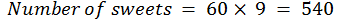7.c)

Number of sweets students got = 60*(20 % of (60)) = 720

Number of sweets Teachers got = 6 *(25% of (60)) = 90

Total number of sweets = 720 + 90 = 810

8.d)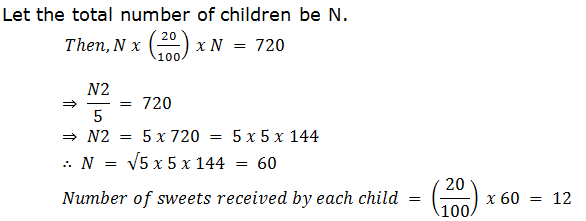9.e)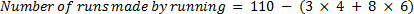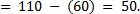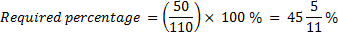10. b)

Let the number be x. Then,

x – 2/5 x = 510

x = (510 * 5)/3 = 850

10% of 850 = 85.

For the other Sections in the Day 3 of the TARGET SBI PLANNER , please check the below given links.

Aspirants can also check the other topics from the SBI CLERK STUDY PLANNER from the link given below. DO bookmark the given link as all the posts will be updated in that page and aspirants can check the daily updates from this page or from the Homepage of Bankersdaily.